# String Standing Waves

For an ideal string of length L which is fixed at both ends, the solutions to the wave equation can take the form of standing waves: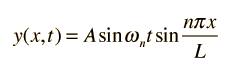This kind of solution can be verified by direct substitution into the wave equation: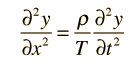Substituting: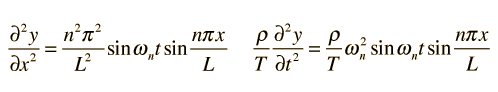These two expressions are equal for all values of x and t provided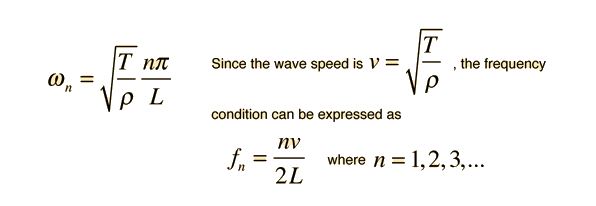Fundamental mode String harmonics
Index

Wave concepts

 HyperPhysics***** Sound R Nave
Go Back

# Fourier Series

If a continuous function f(t) is periodic with period T, then it may be approximated arbitrarily well by a Fourier series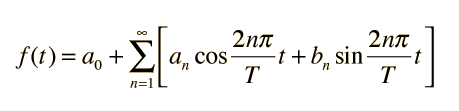where the coefficients are given by: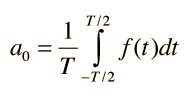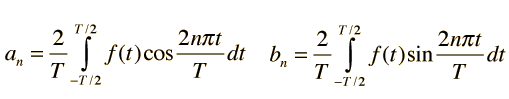Index

Wave concepts

 HyperPhysics***** Sound R Nave
Go Back